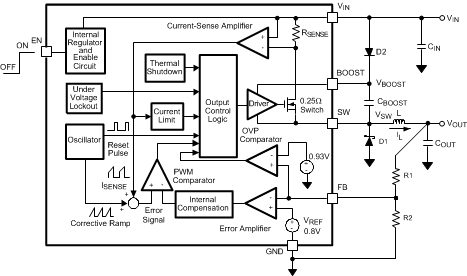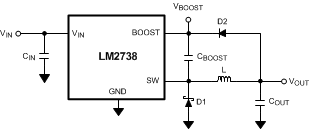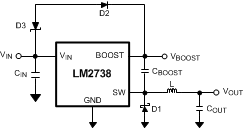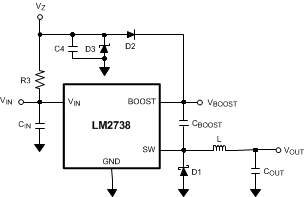SNVS556C April   2008  – January 2016

PRODUCTION DATA.

1. Features
2. Applications
3. Description
4. Revision History
5. Pin Configuration and Functions
6. Specifications
7. Detailed Description
1. 7.1 Overview
2. 7.2 Functional Block Diagram
3. 7.3 Feature Description
4. 7.4 Device Functional Modes
8. Application and Implementation
1. 8.1 Application Information
2. 8.2 Typical Applications
1. 8.2.1  LM2738X Circuit Example 1
1. 8.2.1.1 Design Requirements
2. 8.2.1.2 Detailed Design Procedure
3. 8.2.1.3 Application Curve
2. 8.2.2  LM2738X Circuit Example 2
3. 8.2.3  LM2738X Circuit Example 3
4. 8.2.4  LM2738X Circuit Example 4
5. 8.2.5  LM2738X Circuit Example 5
6. 8.2.6  LM2738Y Circuit Example 6
7. 8.2.7  LM2738Y Circuit Example 7
8. 8.2.8  LM2738Y Circuit Example 8
9. 8.2.9  LM2738Y Circuit Example 9
10. 8.2.10 LM2738Y Circuit Example 10
9. Power Supply Recommendations
10. 10Layout
1. 10.1 Layout Guidelines
2. 10.2 Layout Example
3. 10.3 Thermal Considerations
1. 10.3.1 Silicon Junction Temperature Determination Methods
11. 11Device and Documentation Support
1. 11.1 Device Support
2. 11.2 Documentation Support
3. 11.3 Community Resources
4. 11.4 Trademarks
5. 11.5 Electrostatic Discharge Caution
6. 11.6 Glossary
12. 12Mechanical, Packaging, and Orderable Information

• NGQ|8
• DGN|8
• DGN|8

## 7 Detailed Description

### 7.1 Overview

The LM2738 is a constant frequency PWM buck regulator device that delivers a 1.5-A load current. The regulator has a preset switching frequency of either 550 kHz (LM2738Y) or 1.6 MHz (LM2738X). These high frequencies allow the LM2738 to operate with small surface-mount capacitors and inductors, resulting in DC-DC converters that require a minimum amount of board space. The LM2738 is internally compensated, so it is simple to use and requires few external components. The LM2738 uses current-mode control to regulate the output voltage.

The LM2738 supplies a regulated output voltage by switching the internal NMOS control switch at constant frequency and variable duty cycle. A switching cycle begins at the falling edge of the reset pulse generated by the internal oscillator. When this pulse goes low, the output control logic turns on the internal NMOS control switch. During this on time, the SW pin voltage (VSW) swings up to approximately VIN, and the inductor current (IL) increases with a linear slope. IL is measured by the current-sense amplifier, which generates an output proportional to the switch current. The sense signal is summed with the regulator’s corrective ramp and compared to the error amplifier’s output, which is proportional to the difference between the feedback voltage and VREF. When the PWM comparator output goes high, the output switch turns off until the next switching cycle begins. During the switch off-time, inductor current discharges through Schottky diode D1, which forces the SW pin to swing below ground by the forward voltage (VD) of the catch diode. The regulator loop adjusts the duty cycle (D) to maintain a constant output voltage. See Functional Block Diagram and Figure 25.Figure 25. LM2738 Waveforms of SW Pin Voltage and Inductor Current

### 7.2 Functional Block Diagram### 7.3 Feature Description

#### 7.3.1 Boost Function

Capacitor CBOOST and diode D2 in Figure 26 are used to generate a voltage VBOOST. VBOOST – VSW is the gate-drive voltage to the internal NMOS control switch. To properly drive the internal NMOS switch during its on time, VBOOST must be at least 2.5 V greater than VSW. TI recommends that VBOOST be greater than 2.5 V above VSW for best efficiency. VBOOST – VSW must not exceed the maximum operating limit of 5.5 V. For best performance, see Equation 1.

Equation 1. 5.5 V > VBOOST – VSW > 2.5 V

When the LM2738 starts up, internal circuitry from the BOOST pin supplies a maximum of 20 mA to CBOOST. This current charges CBOOST to a voltage sufficient to turn the switch on. The BOOST pin continues to source current to CBOOST until the voltage at the feedback pin is greater than 0.76 V.

There are various methods to derive VBOOST:

1. From the input voltage (3 V < VIN < 5.5 V)
2. From the output voltage (2.5 V < VOUT < 5.5 V)
3. From an external distributed voltage rail (2.5 V < VEXT < 5.5 V)
4. From a shunt or series Zener diode

As seen on the Functional Block Diagram, capacitor CBOOST and diode D2 supply the gate-drive voltage for the NMOS switch. Capacitor CBOOST is charged via diode D2 by VIN. During a normal switching cycle, when the internal NMOS control switch is off (TOFF) (refer to Figure 25), VBOOST equals VIN minus the forward voltage of D2 (VFD2), during which the current in the inductor (L) forward biases the Schottky diode D1 (VFD1). Therefore the voltage stored across CBOOST is Equation 2:

Equation 2. VBOOST – VSW = VIN – VFD2 + VFD1

When the NMOS switch turns on (TON), the switch pin rises to Equation 3:

Equation 3. VSW = VIN – (RDSON × IL),

forcing VBOOST to rise, thus reverse biasing D2. The voltage at VBOOST is then Equation 4:

Equation 4. VBOOST = 2 VIN – (RDSON × IL) – VFD2 + VFD1

which is approximately 2 VIN – 0.4 V for many applications. Thus the gate-drive voltage of the NMOS switch is approximately VIN – 0.2 V.

An alternate method for charging CBOOST is to connect D2 to the output as shown in Figure 26. The output voltage must be between 2.5 V and 5.5 V so that proper gate voltage is applied to the internal switch. In this circuit, CBOOST provides a gate-drive voltage that is slightly less than VOUT.Figure 26. VOUT Charges CBOOST

In applications where both VIN and VOUT are greater than 5.5 V, or less than 3 V, CBOOST cannot be charged directly from these voltages. If VIN and VOUT are greater than 5.5 V, CBOOST can be charged from VIN or VOUT minus a Zener voltage by placing a Zener diode D3 in series with D2, as shown in Figure 27. When using a series Zener diode from the input, ensure that the regulation of the input supply does not create a voltage that falls outside the recommended VBOOST voltage.

(VINMAX – VD3) < 5.5 V

(VINMIN – VD3) > 2.5 VFigure 27. Zener Reduces Boost Voltage from VIN

An alternative method is to place the Zener diode D3 in a shunt configuration as shown in Figure 28. A small 350-mW to 500-mW 5.1-V Zener in a SOT-23 or SOD package can be used for this purpose. A small ceramic capacitor such as a 6.3-V, 0.1-µF capacitor (C4) must be placed in parallel with the Zener diode. When the internal NMOS switch turns on, a pulse of current is drawn to charge the internal NMOS gate capacitance. The 0.1-µF parallel shunt capacitor ensures that the VBOOST voltage is maintained during this time.Figure 28. Boost Voltage Supplied from the Shunt Zener on VIN

Resistor R3 must be selected to provide enough RMS current to the Zener diode (D3) and to the BOOST pin. A recommended choice for the Zener current (IZENER) is 1 mA. The current IBOOST into the BOOST pin supplies the gate current of the NMOS control switch and varies typically according to the formula in Equation 5 for the X version:

Equation 5. IBOOST = 0.56 × (D + 0.54) × (VZENER – VD2) mA

IBOOST can be calculated for the Y version using Equation 6:

Equation 6. IBOOST = 0.22 × (D + 0.54) × (VZENER – VD2) µA

where

• D is the duty cycle
• VZENER and VD2 are in volts
• IBOOST is in milliamps
• VZENER is the voltage applied to the anode of the boost diode (D2)
• VD2 is the average forward voltage across D2

The formula for IBOOST in Equation 6 gives typical current. For the worst case IBOOST, increase the current by 40%. In that case, the worst case boost current is Equation 7:

Equation 7. IBOOST-MAX = 1.4 × IBOOST

R3 is then given by Equation 8:

Equation 8. R3 = (VIN – VZENER) / (1.4 × IBOOST + IZENER)

For example, using the X-version let VIN = 10 V, VZENER = 5 V, VD2 = 0.7 V, IZENER = 1 mA, and duty cycle
D = 50%. Then Equation 9 and Equation 10:

Equation 9. IBOOST = 0.56 × (0.5 + 0.54) × (5 – 0.7) mA = 2.5 mA
Equation 10. R3 = (10 V – 5 V) / (1.4 × 2.5 mA + 1 mA) = 1.11 kΩ

#### 7.3.2 Soft-Start

This function forces VOUT to increase at a controlled rate during start-up. During soft-start, the error amplifier’s reference voltage ramps from 0 V to its nominal value of 0.8 V in approximately 600 µs. This forces the regulator output to ramp up in a more linear and controlled fashion, which helps reduce in-rush current.

#### 7.3.3 Output Overvoltage Protection

The overvoltage comparator compares the FB pin voltage to a voltage that is 16% higher than the internal reference VREF. Once the FB pin voltage goes 16% above the internal reference, the internal NMOS control switch is turned off, which allows the output voltage to decrease toward regulation.

#### 7.3.4 Undervoltage Lockout

Undervoltage lockout (UVLO) prevents the LM2738 from operating until the input voltage exceeds 2.7 V (typical).

The UVLO threshold has approximately 400 mV of hysteresis, so the part operates until VIN drops below 2.3 V (typical). Hysteresis prevents the part from turning off during power up if the VIN ramp-up is non-monotonic.

#### 7.3.5 Current Limit

The LM2738 uses cycle-by-cycle current limiting to protect the output switch. During each switching cycle, a current limit comparator detects if the output switch current exceeds 2.9 A (typical), and turns off the switch until the next switching cycle begins.

#### 7.3.6 Thermal Shutdown

Thermal shutdown limits total power dissipation by turning off the output switch when the device junction temperature exceeds 165°C. After thermal shutdown occurs, the output switch doesn’t turn on until the junction temperature drops to approximately 150°C.

### 7.4 Device Functional Modes

#### 7.4.1 Enable Pin and Shutdown Mode

The LM2738 has a shutdown mode that is controlled by the enable pin (EN). When a logic low voltage is applied to EN, the part is in shutdown mode, and its quiescent current drops to typically 400 nA. The voltage at this pin must never exceed VIN + 0.3 V.